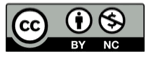# Python 的集合（set）类型

2016-10-10## 概念

``````>>> set([1, 1, 1, 1, 2, 3])
set([1, 2, 3])  #重复元素被排除
>>> set([3, 2, 1])
set([1, 2, 3])  #无序的集合
>>>
``````

## set类型的创建

set类型还有一种标准的表示法是 `set([…])` ，例如

``````>>> {'a','b'}
set(['a', 'b'])
>>>
``````

## set添加元素

``````>>> A = {'a','b'}
>>> A
set(['a', 'c', 'b'])
>>>
``````

## set更新元素

set类型有四个内置update函数用来给set添加元素，对应于Python 的集合运算中的4种运算

## 并集更新

``````>>> A = {'a','b'}
>>> A.update({'c', 'd'})    #iterable的参数都可以传递
>>> A
set(['a', 'c', 'b', 'd'])
>>>
``````

``````>>> A = {'a','b'}
>>> A |= {'c', 'd'}
>>> A
set(['a', 'c', 'b', 'd'])
>>>
``````

## 交集更新

``````>>> A = {'a','b'}
>>> A.intersection_update({'b', 'd'})   #iterable的参数都可以传递
>>> A
set(['b'])
>>>
``````

``````>>> A = {'a','b'}
>>> A &= {'b', 'd'}
>>> A
set(['b'])
>>>
``````

## 差集更新

``````>>> A = {'a','b'}
>>> A.difference_update({'b', 'd'})   #iterable的参数都可以传递
>>> A
set(['a'])
>>>
``````

``````>>> A = {'a','b'}
>>> A -= {'b', 'd'}
>>> A
set(['a'])
>>>
``````

## 对称差集更新

``````>>> A = {'a','b'}
>>> A.symmetric_difference_update({'b', 'd'})   #iterable的参数都可以传递
>>> A
set(['a', 'd'])
>>>
``````

``````>>> A = {'a','b'}
>>> A ^= {'b', 'd'}
>>> A
set(['a', 'd'])
>>>
``````

## set移除元素

remove函数: set类型有好几个内置函数用来从set中移除已有的元素，首先是remove函数，如果remove的元素不存在，会抛出 KeyError 异常。

``````>>> A = {'a','b'}
>>> A.remove('c')
Traceback (most recent call last):
File "<pyshell#65>", line 1, in <module>
A.remove('c')
KeyError: 'c'
>>>
``````

``````>>> A = {'a','b'}
>>> A
set(['a', 'b'])
>>>
``````

## pop函数

``````>>> A = {'a','b'}
set(['a', 'b'])
>>> A.pop()
'a'
>>> A.pop()
'b'
>>> A.pop()
Traceback (most recent call last):
File "<pyshell#71>", line 1, in <module>
A.pop()
KeyError: 'pop from an empty set'
>>>
``````

set的内置函数 clear 用来清空set

``````>>> A = {'a','b'}
set(['a', 'b'])
>>> A.clear()
>>> A
set([])
>>>
``````

## set comprehension

``````>>> {i for i in range(10)}
set([0, 1, 2, 3, 4, 5, 6, 7, 8, 9])
>>>
``````

## 精彩评论